## Systems of Linear Inequalities

### Learning Outcomes

• Identify solutions to systems of linear inequalities

## Graph a System of Two Inequalities

Remember from the module on graphing that the graph of a single linear inequality splits the coordinate plane into two regions. On one side lie all the solutions to the inequality. On the other side, there are no solutions. Consider the graph of the inequality $y<2x+5$.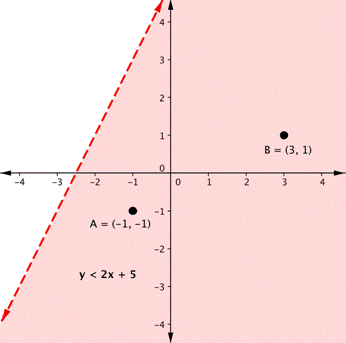The dashed line is $y=2x+5$. Every ordered pair in the shaded area below the line is a solution to $y<2x+5$, as all of the points below the line will make the inequality true. If you doubt that, try substituting the x and y coordinates of Points A and B into the inequality; you will see that they work. So, the shaded area shows all of the solutions for this inequality.

The boundary line divides the coordinate plane in half. In this case, it is shown as a dashed line as the points on the line do not satisfy the inequality. If the inequality had been $y\leq2x+5$, then the boundary line would have been solid.

Now graph another inequality: $y>−x$. You can check a couple of points to determine which side of the boundary line to shade. Checking points M and N yield true statements. So, we shade the area above the line. The line is dashed as points on the line are not true.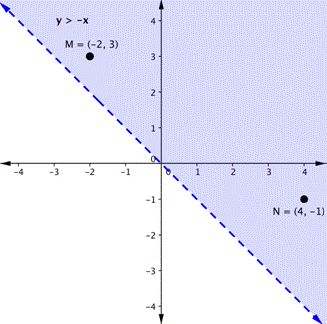To create a system of inequalities, you need to graph two or more inequalities together. Let us use $y<2x+5$ and $y>−x$ since we have already graphed each of them.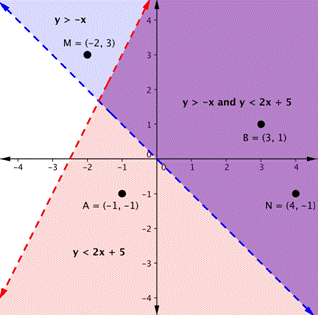The purple area shows where the solutions of the two inequalities overlap. This area is the solution to the system of inequalities. Any point within this purple region will be true for both $y>−x$ and $y<2x+5$.

In the following video examples, we show how to graph a system of linear inequalities and define the solution region.

In the next section, we will see that points can be solutions to systems of equations and inequalities. We will verify algebraically whether a point is a solution to a linear equation or inequality.

## Determine Whether an Ordered Pair is a Solution to a System of Linear Inequalities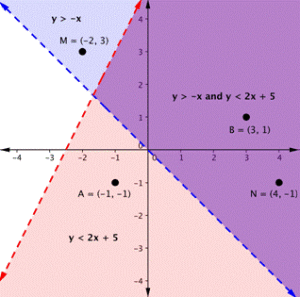On the graph above, you can see that the points B and N are solutions for the system because their coordinates will make both inequalities true statements.

In contrast, points M and A both lie outside the solution region (purple). While point M is a solution for the inequality $y>−x$ and point A is a solution for the inequality $y<2x+5$, neither point is a solution for the system. The following example shows how to test a point to see whether it is a solution to a system of inequalities.

### Example

Is the point $(2, 1)$ a solution of the system $x+y>1$ and $2x+y<8$?

Here is a graph of the system in the example above. Notice that $(2, 1)$ lies in the purple area which is the overlapping area for the two inequalities.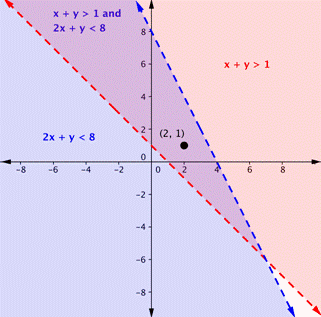### Example

Is the point $(2, 1)$ a solution of the system $x+y>1$ and $3x+y<4$?

Here is a graph of the system above. Notice that $(2, 1)$ is not in the purple area which is the overlapping area; it is a solution for one inequality (the red region), but it is not a solution for the second inequality (the blue region).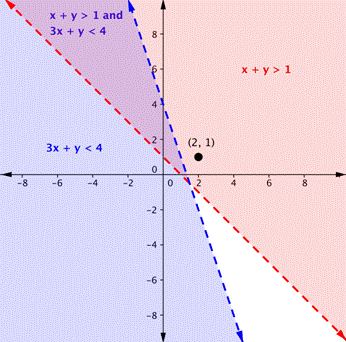In the following video, we show another example of determining whether a point is in the solution of a system of linear inequalities.

As shown above, finding the solutions of a system of inequalities can be done by graphing each inequality and identifying the region they share. Below, you are given more examples that show the entire process of defining the region of solutions on a graph for a system of two linear inequalities.  The general steps are outlined below:

• Graph each inequality as a line and determine whether it will be solid or dashed.
• Determine which side of each boundary line represents solutions to the inequality by testing a point on each side.
• Shade the region that represents solutions for both inequalities.

## Systems with No Solutions

In the next example, we will show the solution to a system of two inequalities whose boundary lines are parallel to each other.  When the graphs of a system of two linear equations are parallel to each other, we found that there was no solution to the system.  We will get a similar result for the following system of linear inequalities.

### Examples

Graph the system $\begin{array}{c}y\ge2x+1\\y\lt2x-3\end{array}$

## Summary

• Solutions to systems of linear inequalities are entire regions of points.
• You can verify whether a point is a solution to a system of linear inequalities in the same way you verify whether a point is a solution to a system of equations.
• Systems of inequalities can have no solutions when boundary lines are parallel.